Math & Beyond
Pattern Recognition
9.4k views

 1 Introduction 2 What is a Pattern? 3 Types of Patterns 4 Example Problems 5 Practice Questions 6 Summary 7 Frequently Asked Questions (FAQs) 8 External References

30 December 2020

## Introduction

We see ‘Patterns’ all around us all the time.

There is a pattern in day and night. There is a pattern in seasons. There is a pattern in the petals of the flower, there is a pattern in the ripples of the water pond.

In this blog, we will learn how to decode this pattern.

## What is a Pattern?

A pattern is a series or sequence that repeats. Math Patterns are sequences that repeat based on a rule, and a rule is a set way to calculate or solve a problem.

We will work on some examples step by step to find out a way to get to these rules. Download the PDF to understand Pattern Recognition.

## Types of Patterns

• Letter Pattern

• Number Pattern

• Geometric/Shape Pattern

## Example Problems

Example 1: What is the missing letter in the series?(A) U             (B) V             (C) W             (D) None of these

Step 1. Write the sequence separately and observe the letter closely. You will notice that the letters are not in order. There are missing letters in between.

Step 2.  Write those missing letters.
P   Q   R   S   T  __    X

Step 3.  Now if you observe the letters, it is very clear that the consecutive letters are missing if we go by the universal sequence of 26 letters of English Alphabets. And the letter next to the consecutive letter is part of the sequence.

Step 4. So in the sequence of English Alphabets ‘U’ is the consecutive letter of ‘T’ or ‘U’ comes next to ‘T '.So we will drop ‘U’. And the letter next to ‘U’ ie ‘V’ will become part of the series or sequence.

Step 5. Select the option which has ‘V’ as the answer.

 (B) V

Example: Find the missing numbers to complete the given number series.
16, 25, 36, ?, ?, 81, 100

(A) 49, 64          (B) 64, 49            (C) 49, 81               (D) 81, 64

Step 1. Write the sequence separately and observe the numbers.

Step 2. Check if the difference between 25 and 16, 36 and 25 , 100 and 81 is the same. On calculating we get 9,11 and 19. So this rule is ruled out. As this difference is neither same nor in any particular sequence.

Step 3.  Check if these numbers have common multiples. NO. So this is also ruled out.

Step 4. Check the factors of these numbers
$$16= 4*4$$
$$25=5*5$$
$$36=6*6$$
?
?

$$81=9*9$$
$$100=10*10$$
Here we see a pattern. The given numbers are squares of consecutive numbers like 4,5,6 ?? 9 and 10. We cracked the rule.

Step 5. So the missing numbers are squares of 7 and 8.
$$7*7= 49$$
$$8*8=64$$

Step 6. Look for an option 49,64. Ie (A).

 (A) 49,64

Example: Complete the figure pattern given below.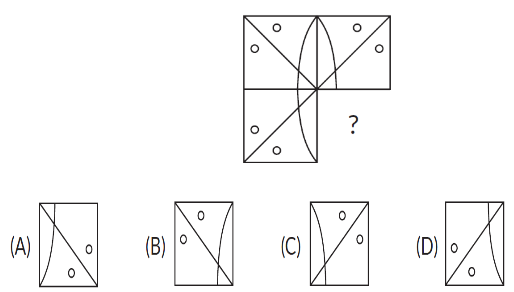Step 1. Let us name the 3 tiles in this figure as  ‘W’ ‘X’ ‘Y’ and we have to find the 4th tile which fits in this pattern. Let us name that as ‘Z’.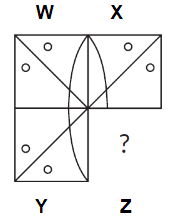Step 2. Check if X is just a repetition of W taken as it is. It’s not.

Step 3. Check if W is rotated right or left, does it become X. No.

Step 4. Check if W and X are mirror images of each other. Yes. So we cracked the rule. Each tile in the figure is a mirror image of its adjoining tile.

Step 5. Look for an option that is a mirror image of Y. It is option (A).

 (A)

## Practice Questions

Question 1: Find the missing number.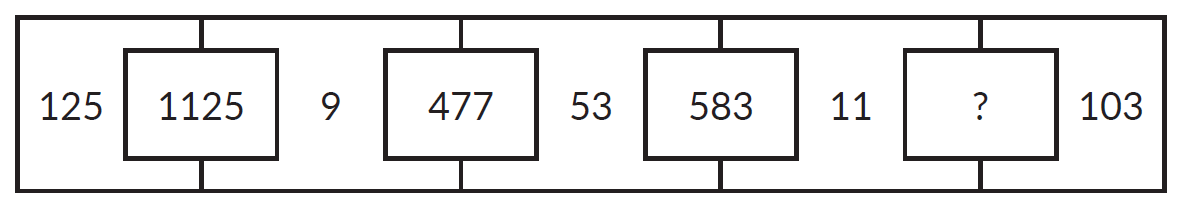(A) 1313       (B) 1331        (C) 1133        (D) 3131

Question 2: What comes next in the given pattern?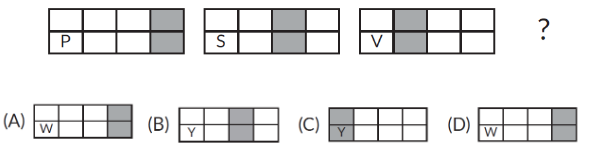Question 3: Spot the incorrect option.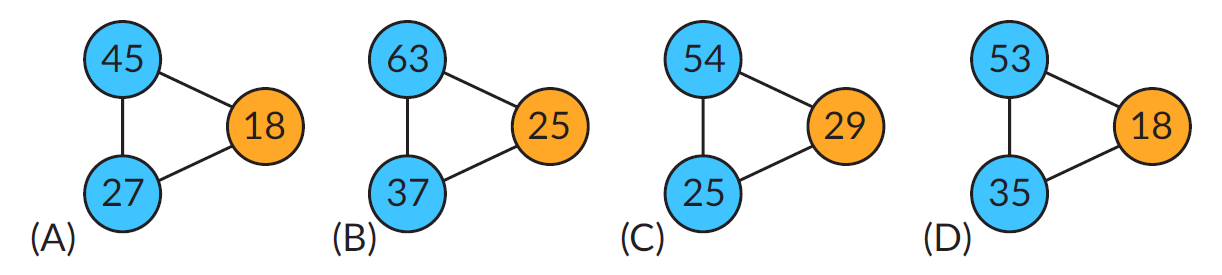Question 4: Which figure comes next in the given figure pattern?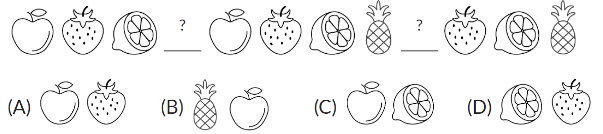Question 5: Find the missing numbers, if a certain rule is followed in the figure.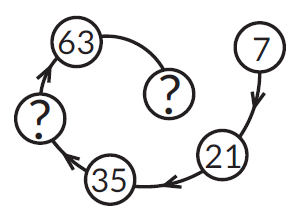(A) 49, 77              (B) 77, 63                     (C) 63, 49             (D) 70, 42

 1. (C)     2. (C)     3. (B)     4. (B)     5. (A)

## Summary

Learning patterns enhances our capability to observe patterns. Observing a pattern pushes us to think and identify the rule which can continue the pattern.

Pattern questions are part of most of the grades from KG to Class 12. The competitive exams also have an aptitude section which has most of the questions related to patterns.

Patterns go a long way in building our mental maths which in turn helps in various problem-solving situations.

Cuemath, a student-friendly mathematics and coding platform, conducts regular Online Classes for academics and skill-development, and their Mental Math App, on both iOS and Android, is a one-stop solution for kids to develop multiple skills. Understand the Cuemath fee structure and sign up for a free trial.

## How to teach patterns in math?

• Do simple exercises at home like putting the beads in the string, take the building blocks of different shapes and sizes and try to keep them in a particular sequence creating a series, set a pattern in the meals you take during the day. These are small simple ways to introduce patterns.

• For higher classes, make numbers your friend. Know their factors , multiples, which numbers are squares and which are cubes. This will help you to crack number series.

• For letter series , write the letters and try to find the missing letters. Sometimes the questions are based on the numeric value of alphabets. For example ‘A’ =1, ‘B’ =2….. Try to decode if there is some co -relation in this particular format.

• For figure series observe and try to crack the relation between the figures in the series. For example, at what intervals they repeat, if they repeat in rotated or mirror image form or their original form.

## How to draw geometric patterns in math?

To draw geometric patterns you should use a grid as illustrated in the image below.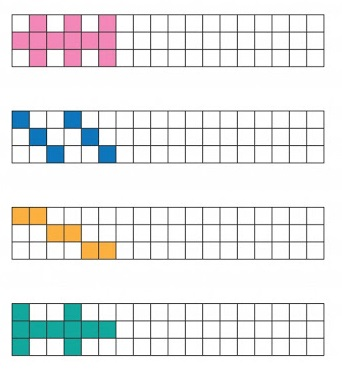Drawing patterns in a grid is very simple. You have to  color one square in a grid and count the number of squares after which it should be repeated. Then again count the same number of squares and colour the square.

This way you will get a pattern or a sequence that repeats. Pattern is nothing but a sequence that repeats.

-Written by Richa Khare, Cuemath Teacher

Related Articles